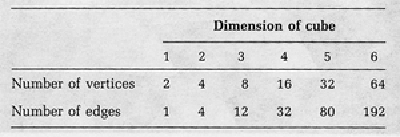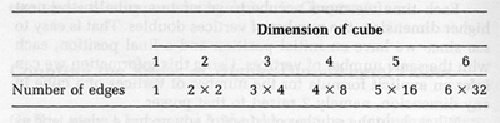Counting the Edges Of Higher-Dimensional Cubes

On first view, a hypercube in the plane can be a confusing pattern of lines. Images of cubes from still higher dimensions become almost kaleidoscopic. One way to appreciate the structure of such objects is to analyze lower-dimensional building blocks.

We know that a square has 4 vertices, 4 edges, and 1 square face. We can build a model of a cube and count its 8 vertices, 12 edges, and 6 squares. We know that a four-dimensional hypercube has 16 vertices, but how many edges and squares and cubes does it contain? Shadow projections will help answer these questions, by showing patterns that lead us to formulas for the number of edges and squares in a cube of any dimension whatsoever.

It is helpful to think of cubes as generated by lower-dimensional cubes in motion. A point in motion generates a segment; a segment in motion generates a square; a square in motion generates a cube; and so on. From this progression, a pattern develops, which we can exploit to predict the numbers of vertices and edges.

Each time we move a cube to generate a cube in the next higher dimension, the number of vertices doubles. That is easy to see since we have an initial position and a final position, each with the same number of vertices. Using this information we can infer an explicit formula for the number of vertices of a cube in any dimension, namely 2 raised to that power.

What about the number of edges? A square has 4 edges, and as it moves from one position to the other, each of its 4 vertices traces out an edge. Thus we have 4 edges on the initial square, 4 on the final square, and 4 traced out by the moving vertices for a total of 12. That basic pattern repeats itself. If we move a figure in a straight line, then the number of edges in the new figure is twice the original number of edges plus the number of moving vertices. Thus the number of edges in a four-cube is 2 times 12 plus 8 for a total of 32. Similarly we find 32 + 32 + 16 = 80 edges on a five-cube and 80 + 80 + 32 = 192 edges on a six-cube.

By working our way up the ladder, we find the number of edges for a cube of any dimension. If we very much wanted to know the number of edges of an n-dimensional cube, we could carry out the procedure for 10 steps, but it would be rather tedious, and even more tedious if we wanted the number of edges of a cube of dimension 101. Fortunately we do not have to trudge through all of these steps because we can find an explicit formula for the number of edges of a cube of any given dimension.

One way to arrive at the formula is to look at the sequence of numbers we have generated arranged in a table.If we factor the numbers in the last row, we notice that the fifth number, 80, is divisible by 5, and the third number, 12, is divisible by 3. In fact, we find that the number of edges in a given dimension is divisible by that dimension.This presentation definitely suggests a pattern, namely that the number of edges of a hypercube of a given dimension is the dimension multiplied by half the number of vertices in that dimension. Once we notice a pattern like this, it can be proved to hold in all dimensions by mathematical induction.

There is another way to determine the number of edges of a cube in any dimension. By means of a general counting argument, we can find the number of edges without having to recognize a pattern. Consider first a three-dimensional cube. At each vertex there are 3 edges, and since the cube has 8 vertices, we can multiply these numbers to give 24 edges in all. But this procedure counts each edge twice, once for each of its vertices. Therefore the correct number of edges is 12, or three times half the number of vertices. The same procedure works for the four-dimensional cube. Four edges emanate from each of the 16 vertices, for a total of 64, which is twice the number of edges in the four-cube.

In general, if we want to count the total number of edges of a cube of a certain dimension, we observe that the number of edges from each vertex is equal to the dimension of the cube n, and the total number of vertices is 2 raised to that dimension, or 2n. Multiplying these numbers together gives n × 2n, but this counts every edge twice, once for each of its endpoints. It follows that the correct number of edges of a cube of dimension n is half of this number, or n × 2n-1. Thus the number of vertices of a seven-cube is 27 = 128, while the number of edges in a seven-cube is 7 × 26 = 7 × 64 = 448.